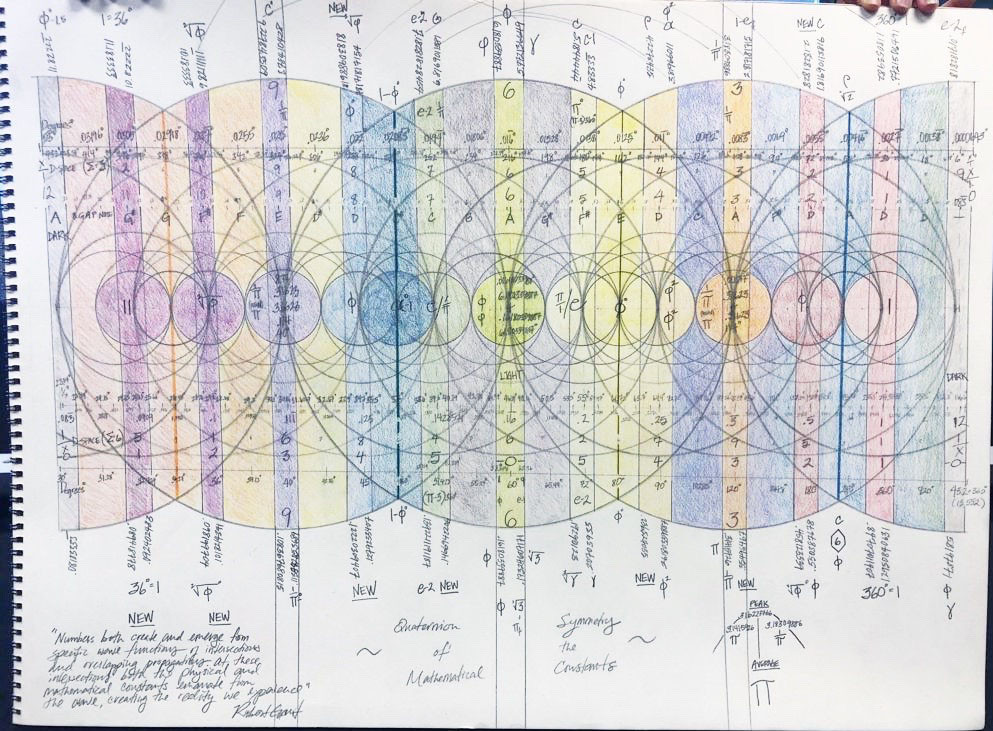Search

# The Mystery of the Constants

My latest work on the constants...The math behind the separation of light from darkness...and the separation and extraction of matter and energy from the vacuum...The Mathematical Constants

First, it is amazing to me how consciousness expands when we ask the deeper questions...and the knowledge that comes always has an artistic quality...not something I have ever noticed before with mathematics...but then again, the universe is beautiful so math must also have a beautiful expression.

I started the above with a simple test using x/1 and 1/x symmetry.  What emerged is the following...1.6180339887... and .6180339887...this is the crossover point or center of the chart.

These numbers serve to “frame” the integer number 6 (the hexagon and esoterically significant).....the wave is separated at 3.1623/.31623 which happens to be exactly the average of Pi (3.1415926 and its 1/x equivalent .31830988) and likewise 10^0.5.  Here, like Phi at the center, the numbers converge and overlap at this nodal position.

The macro explanation of the illustration is as follows:

1. Based on this research, numbers BOTH create and emerge from wave propagations of electromagnetism. This is very simple ‘doubling math’.....every circle creates two smaller circles in the form of a vesica pisces within it, and this pattern continues into infinity. This repeating (and balanced) pattern continues and transmutes into the reality we experience everyday through successive propagations and presumably higher energy amplitudes.

2. Out of the nodal points of geometrical overlaps (intersections) the mathematical and physical constants emerge (emanate) along the wave of numbers (incidentally the Periodic Wave of Elements also emerge in similar fashion).  This phenomenon manifests as crossover zones when oscillating between x/1 and 1/x representations. Electricity, magnetism, radiation and gravity thus result from these wave (and numerical) propagations.

3. The image depicts many critical zones with differing references to Phi as well as the other fundamental constants (Phi (decimal), Phi (degrees), etc) as well as their quaternion and octonion counterparts (mirrored reflections and absorptions). The first of these separations occur at center on the conjunction of Phi and 1/Phi. This action is “framed” by Phi (1.618), Phi-1, 3^.5 (1.732), and Gamma (0.5772).

4. Musical notes (including their associated polygons (2D) and polyhedra (3D) as well as those higher dimensional geometries) and are depicted in cycles per second (Hz), emerging from the conjunctions of intersection as a result of ‘overlapping’ waves.

5. Emanating outward from center, the next critical wave interference is appropriately framed by the Pi fractal (.14) and Euler fractal (.71) create light. Euler thus serves to shape the vacuum and growth of the wave propagation, with Pi forming the spirality of the wave; always working together with Euler to shape light and matter by framing the conditions surrounding matter and vacuum. From the continual interaction of these mathematical and physical constants related to radiation emerges light, matter, and energy; and out of gravity emanates time, mass and darkness (vacuum). Light begins it reflection/absorption from near the center of the wave at 5.184 (186 degrees (C))(which also just happens to be the slope angle of the Great Pyramid). The backdrop to this convergence is the blackness of the vacuum.  The western framing of vacuum happens at 6.8 (and 1.68 (Euler/Phi = Grant’s Constant governing the relationship of mass and time). The mirror reflection of light simultaneously is dark and the emergence of Time itself. Grant’s Constant determines time (periodicity) and is equivalent to energy/radiation.

6. The next outer framing is bounded by Phi in degrees 162 degrees and its mirror and 37 degrees which is Euler (2.71828 in 1/x calculation).  Each subsequent outer framing both converges and diverges simultaneously like concave and convex lens to concentrate and disperse energy to separate light from darkness...separating nothingness from matter propagating from center toward the outer edges of the chart.

7. The next major framing happens at integer number 9 and integer number 3.  At 9 and 3, the 1/Pi Constant emerges at the overlapping conjunction of 3.1623 and .31623. This mirror is created in the 1/x and x/1 merger ((averaging) of Pi: 3.1414826 and 3.18309886). This is also a simple “halving” of the wave on both sides of the Phi junction at center where light emerges and simultaneously gives form to matter.

All of the above matches beautifully with the icositetragon (24-sided polygon) and the positioning of the primes and twin primes that also serve to “frame” and “lock” the above interactions and its emergent phenomena further still.Quaternion Symmetry of the Mathematical Constants

Thus, we now know how the separation of light and dark is achieved mathematically emanating from a dynamic wave.  We also understand better how time emerges from this function combined with our observation as well as the likely hypothesis that at each halving of a wave another mirroring Constant (fractal) likewise become an inherent result of the convergence.

Who would have thought that the most simple 1/x and x/1 analysis along with the language of light would combine to reveal the mystery of the constants into ONE unifying mathematical theory? There is yet more work to be done to further confirm and refine our identified patterns so stay tuned...indeed, from this work, one could say that all constants emerge from mirror reflections and wave emanations from the same ONENESS, which reflection is Phi and which absorption is Alpha.

7,086 views1 comment

See All